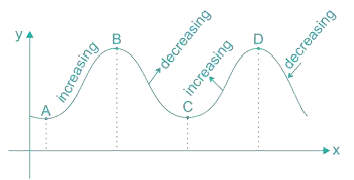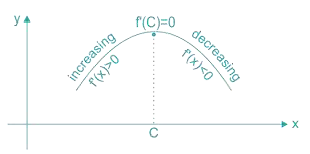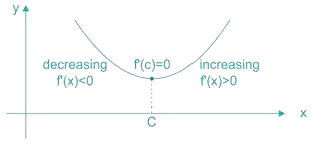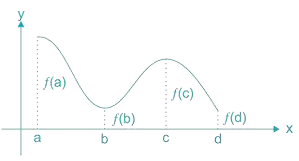Courses

Maxima and Minima Notes | Study Engineering Mathematics - GATE

GATE: Maxima and Minima Notes | Study Engineering Mathematics - GATE

The document Maxima and Minima Notes | Study Engineering Mathematics - GATE is a part of the GATE Course Engineering Mathematics.
All you need of GATE at this link: GATE

Definition of Maxima and Minima

1. The function f is said to have a maximum value in I, if there exists a point c in such that f(c) > f(x), for all x I. The value f(c) is called the maximum value of f(x) in I and the point c is called a point of maximum value of f(x) in I.
2. The function f is said to have a minimum value in I, if there exists a point c in I such that f(c) < f(x), for all x I. The value f(c), in this case, is called the minimum value of f(x) in I and the point c, in this case is called a point of minimum value of
f(x) in I.
3. The function f is said to have an extreme value in I if there exists a point c in I such that f(c) is either maximum value or a minimum value of f(x) in I.
4. The number f(c) in this case, is called an extreme value of f(x) in I and the point c is called an extreme point.Here Point A, C are Local Minima and B, D are Local Maxima.

Notes

1. Concave Downwards indicates Maxima of the function i.e.2. Concave Upwards indicates Minima of the function i.e.3. If f ‘(x) does not change sign as x increases through c, then c is neither a point of Local maxima nor a point of local minima. In fact, such a point is called point of inflection. So the condition for point of inflection is f ‘’(x) = 0
4. Similarly the necessary condition for the existing of either Maxima or Minima is f ‘(x) = 0.

Example 1: Find all the points of local maxima and local minima of the function f given by f(x) = 2x3 - 6x2 + 6x + 5
Solution: Given that f(x) = 2x3 - 6x2 + 6x + 5
f ‘(x) = 6x2 - 12x + 6 = 6(x - 1)2
f ‘(x) = 0 at x = 1
Observe that f ‘(x) > 0 for all x R and in particular f ‘(x) > 0, for values close to 1 and to the left and right of 1.
Hence x = 1 is a point of inflection.

Definition of Local Maxima and Minima

Let f be a function defined on an interval I and c I. Let f be twice differentiable at c.
Then

1. x = c is a point of local maxima if f ‘(c) = 0 and f ‘'(c) < 0. Then the value f(c) is local maximum value of f(x).
2. x = c is a point of local minima if f ‘(c) = 0 and f ‘'(c) > 0. In this case, f(c) is local minimum value of f(x).

Example 2: Find local maximum and local minimum values of the function f given by
f(x) = 3x4 + 4x3 - 12x2 + 12

Solution: f(x) = 3x4 + 4x3 - 12x2 + 12
f ‘(x) = 12x3 + 12x2 – 24x = 12x(x – 1)(x + 2)
f ‘(x) = 0 at
x = 0, x = 1 and x = –2
f ‘'(x) = 36x2 + 24x – 24
f ‘'(0) = –24 < 0
f ‘'(1) = 36 > 0
f ‘'(–2) = 144 – 48 – 24 = 72 > 0
x = 0 is a point of local maxima and local maximum value of f(x) at x = 0 is f(0) = 12 while x = 1 and x = –2 are local minimum points.
Local minimum values are f(1) = 7 and f(–2) = 20

Absolute Maxima and Absolute Minima1. The graph gives a continuous function defined on a closed interval [a, d]. Observe that the function f has a local minima at x = b and local minimum vales is f(b), the function also has a local maxima at x = c and local maximum values is f(c).
2. Also form the graph, it is evident that f has absolute maximum value f(a) and absolute minimum value f(d). Further note that absolute maximum (minimum) value of f(x) is different from local maximum (minimum) value of f(x).
3. This absolute maximum value is nothing but global maxima and absolute minimum value is nothing but global minima.

Method to Find Global Maxima and MinimaStep 1: Find the all critical points of the function f(x) in the interval i.e. find points x where either f ‘(x) = 0 or f is not differentiable.

Step 2: Take the end points of the interval.

Step 3: At all these points (listed in step 1 and step 2) calculate the values of f(x).

Step 4: Identity the maximum and minimum values of f(x) out of the values will be the absolute maximum (greatest) value of f(x) and the minimum (least) value of f(x).

Example 3: Find the absolute maximum and minimum values of a function f(x) given by   f(x) = 2x3 – 15x2 + 36x + 1  in the interval [1, 5]

Solution: f '(x) = 6x2 – 30x + 36 = 6(x – 3)(x – 2)
f '(x) = 0 gives x = 2, x = 3
We shall evaluate the f(x) at x = 2 and x = 3 and at the end points.
f (1) = 24
f(2) = 29
f(3) = 28
f(5) = 56
So absolute maximum value is 56 at x = 5
Absolute minimum value is 24 at x = 1

The document Maxima and Minima Notes | Study Engineering Mathematics - GATE is a part of the GATE Course Engineering Mathematics.
All you need of GATE at this link: GATEUse Code STAYHOME200 and get INR 200 additional OFF Use Coupon CodeEngineering Mathematics

54 docs|21 tests

Track your progress, build streaks, highlight & save important lessons and more!

,

,

,

,

,

,

,

,

,

,

,

,

,

,

,

,

,

,

,

,

,

;